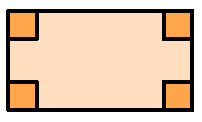### Home > ACC6 > Chapter 1 Unit 10 > Lesson CC2: 1.2.7 > Problem1-128

1-128.

Draw an example of each of the following shapes.

1. rectangle

What are the characteristics of a rectangle?

A rectangle has $4$ sides and $4$ ninety degree angles.1. square

What are the characteristics of a square?

A square has $4$ sides of equal length and $4$ ninety degree angles.

1. parallelogram

What are the characteristics of a parallelogram?

A parallelogram has $4$ sides with two pairs of parallel sides.

1. trapezoid

What are the characteristics of a trapezoid?

A trapezoid is a quadrilateral with at least one pair of parallel sides.

1. scalene triangle

What are the characteristics of a scalene triangle?

A scalene triangle has no congruent sides.

1. right triangle

What are the characteristics of a right triangle?

A right triangle has one right angle.